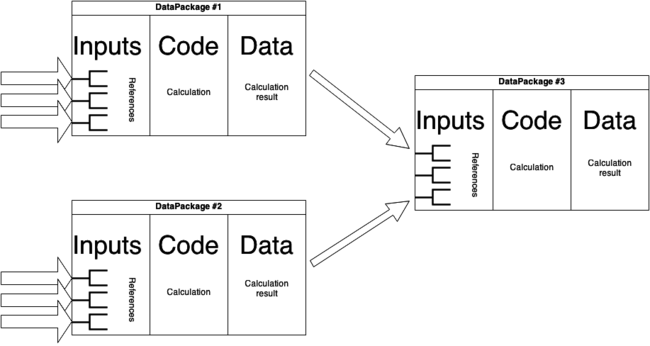# 2+2<4

by Krisztián Fekete

Being precise in describing how we achieve a result is hard.

It is not optional, though. Let’s look at the inequality `2+2<4`.
Since we have learnt `2+2=4` in primary school, the inequality looks strange. It might become true, though, if we extend it with further information–what the units are and what we mean by the + operation.

`2+2<4` might be a short-hand for some mud-making specialists for `2 liters of sand mixed with 2 liters of water gives less than 4 liters of mud`, but no one else except the mud-makers would understand it (we get less than 4 liters of mud, as the sand drinks up some water).

For a result to be meaningful and reproducible, both input (such as 2 liters of sand and 2 liters of water) and operation (such as mixing the two substances) are important to be given explicitly, otherwise we will have not have the expected result (mud with a total volume of less than 4 liters).

In our work we do a lot of data transformations and calculations. Our result is data, our input is also data, our operation is a computer program.
Input, code and output are strongly related to each other (as in the mud example above), so it makes sense to pack them together.

Our input data is usually also a result of some calculations, so we end up with this model:This is a simple dependency graph, where the data is packed together with the code producing it.

When there is no intermediate data (there is only one program), this setup occurs naturally, as it is the easiest thing to do.

For more complex workflows, we have abandoned this model and separated code (version control, github) and data (file servers) and thus have to maintain links between the two (problematic!). As if code likes to live with code and data with data.

To be able to return to sanity (with packages like on the picture) we need

• tools for working with packages consisting of
• data
• code that produces it
• input data references
• to easily reference other packages (thus accessing their data for input)
• to easily re-create a package with updated inputs
• tool to verify packages, that the included code indeed produce the output
• tool to export a whole workflow as a code, so that we can run it in one step
• potentially all of this to work offline without a server